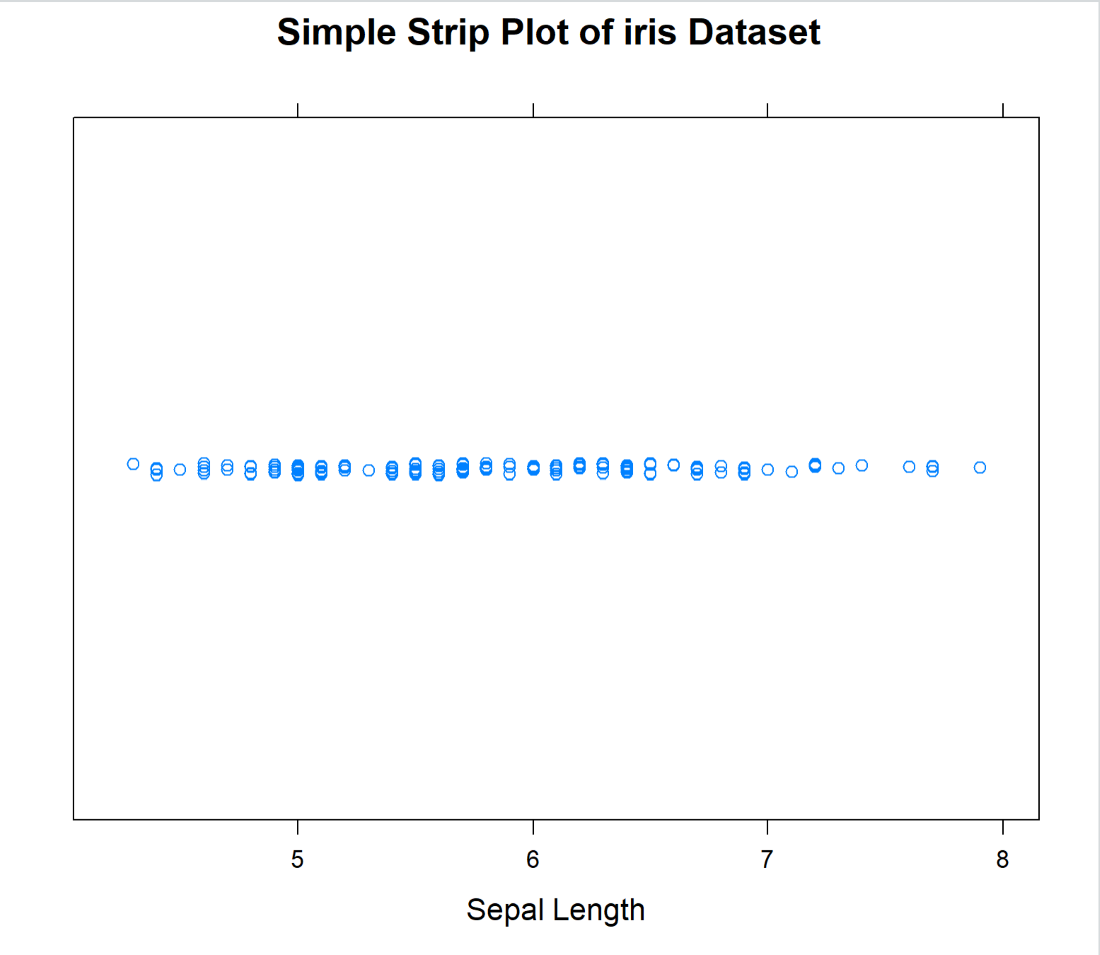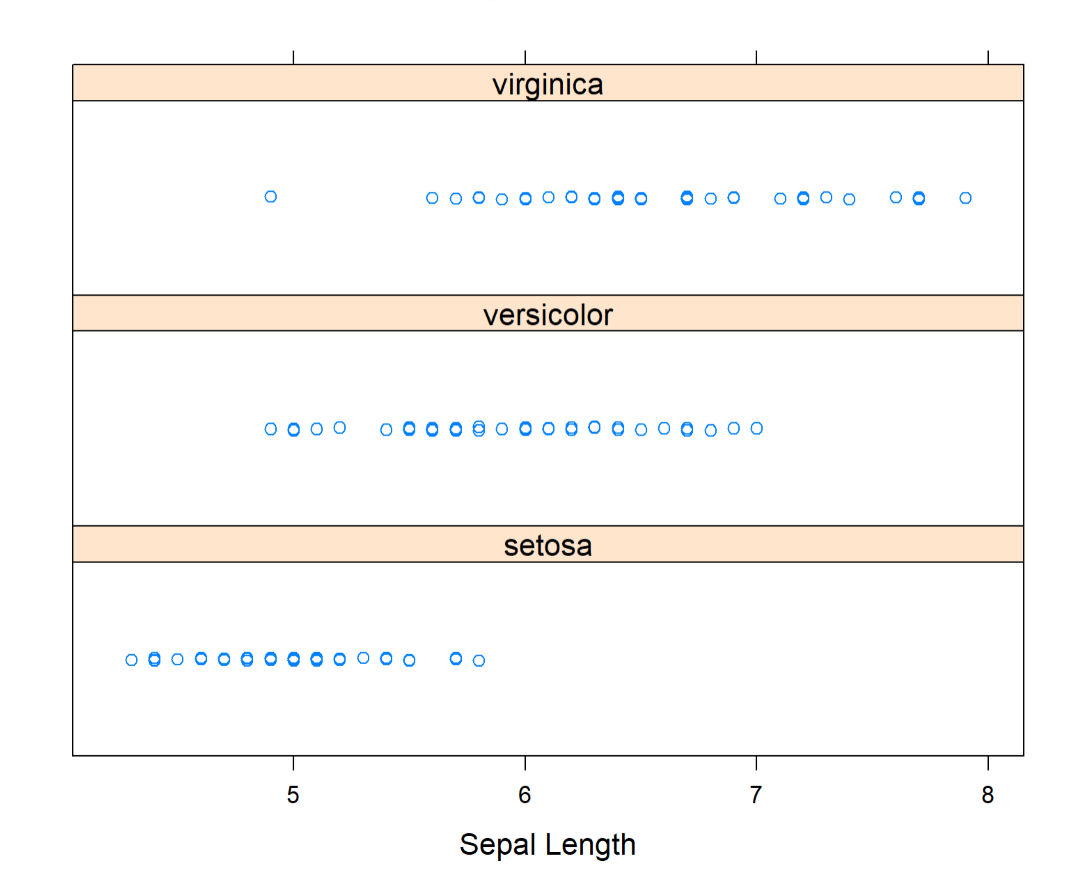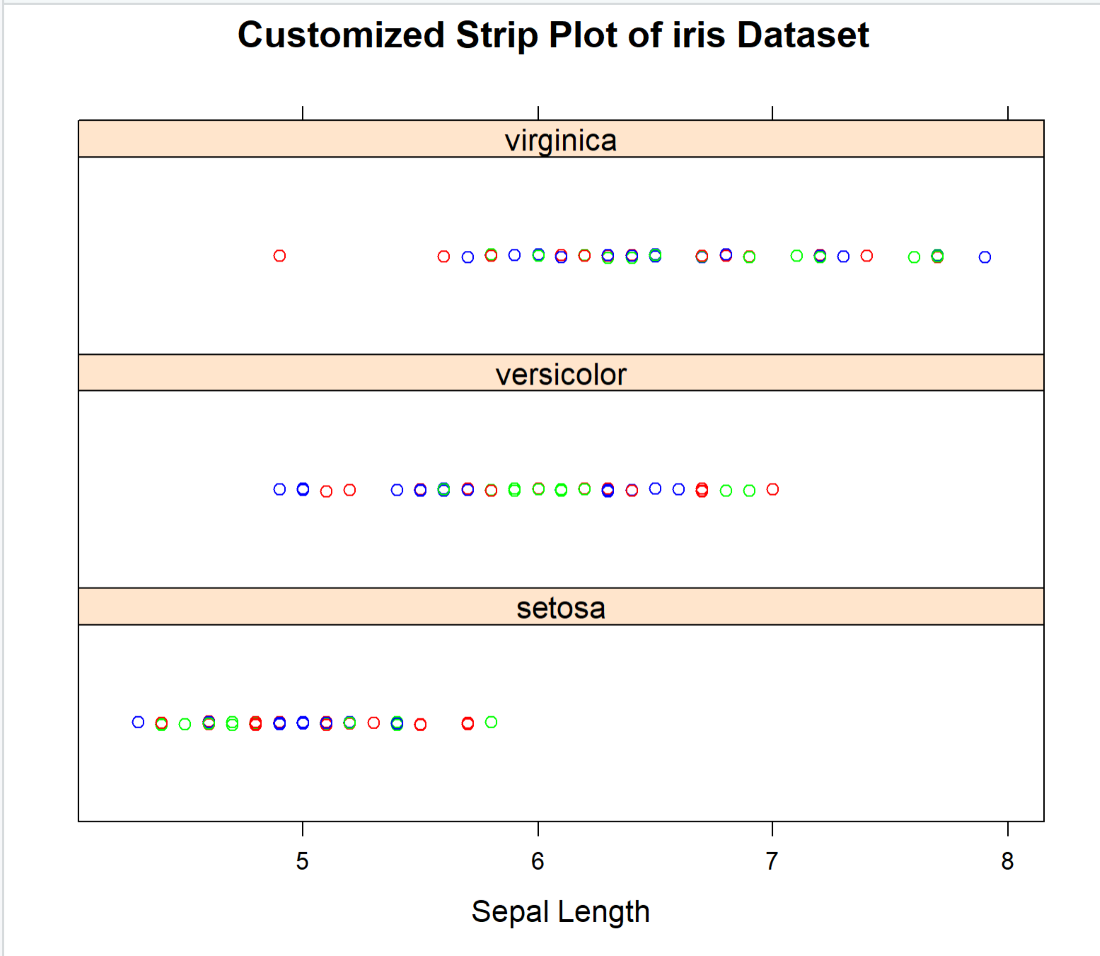GeeksforGeeks App
Open AppBrowser
Continue

# Strip Plots Using Lattice Package in R

In general, Strip Plots are used to plot univariate data. It’s a 2D plot where on X-Axis the response variables are plotted.

These are alternatives to the histogram or density plot. It is typically used to plot small data sets so here we will be using the iris dataset to implement it.

In, R Programming, the Strip Plots can be implemented using the stripplot() function which is present in the lattice package.

Syntax: stripplot(formula,data,jitter,factor,xlab,ylab,main)

where,

• data – data frame that needs to be considered to plot
•  jitter – used to specify whether the values should be jittered or not
• factor – used to specify the amount of jitter
• xlab – used to specify the X-axis label
• main – used to specify the title of the plot

Let’s see the code for Simple Strip Chart. In this, we will be using the iris dataset and plotting the sepal length using stripplot().

## R

 `# Install the required package``install.packages``(``"lattice"``)``# Load the installed package``library``(lattice)`` ` `# Load the default dataset(iris) into current working environment ``data``(iris)`` ` `# Plotting the Simple Strip plot using striplot()``lattice::``stripplot``(~Sepal.Length, data=iris,``                   ``jitter=``TRUE``, xlab=``"Sepal Length"``,``                   ``main=``"Simple Strip Plot of iris Dataset"``)`

Output:We can also plot the Partitioned Strip Plot for each category. Here we are categorizing according to Species column. The code is given below.

## R

 `# Install the required package``install.packages``(``"lattice"``)``# Load the installed package``library``(lattice)`` ` `# Load the default dataset(iris) into current working environment ``data``(iris)`` ` `# Plotting the Partitioned Strip plot using striplot()``lattice::``stripplot``(~Sepal.Length|Species, data=iris,``                   ``jitter=``TRUE``, layout=``c``(1,3),``                   ``xlab=``"Sepal Length"``)`

Output:We can also customize the plot using col parameter. We can add different colors to the plot.

## R

 `# Install the required package``install.packages``(``"lattice"``)``# Load the installed package``library``(lattice)`` ` `# Load the default dataset(iris) into current working environment ``data``(iris)`` ` `# Plotting the Customized Strip plot using striplot()``lattice::``stripplot``(~Sepal.Length|Species, data=iris,``                   ``jitter=``TRUE``,layout=``c``(1,3), xlab=``"Sepal Length"``,``                   ``main=``"Customized Strip Plot of iris Dataset"``,``                   ``col=``c``(``"red"``,``"blue"``,``"green"``))`

Output:My Personal Notes arrow_drop_up